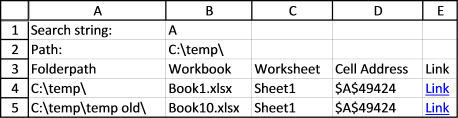Author: Oscar Cronquist Article last updated on August 29, 2017

Search all workbooks in a folder is a popular post, I am happy so many find it useful.

hi thanks for the great macro really makes a hard job much easier, can this be made to search subfolders as well?
thanks
rusl

Is there a way to make the search look in subdirectories as well

This is an example of a search i did in c:\temp on my harddrive.The following vba macro searches workbooks in a folder and subfolders with file extensions *.xls, *.xlsx and *.xlsm.

```Public WS As Worksheet

Sub SearchWKBooksSubFolders(Optional Folderpath As Variant, Optional Str As Variant)
Dim myfolder As String
Dim a As Single
Dim sht As Worksheet
Dim Lrow As Single
Dim Folders() As String
Dim Folder As Variant
ReDim Folders(0)

If IsMissing(Folderpath) Then
Set WS = Sheets.Add

With Application.FileDialog(msoFileDialogFolderPicker)
.Show
myfolder = .SelectedItems(1) & "\"
End With

Str = Application.InputBox(prompt:="Search string:", Title:="Search all workbooks in a folder", Type:=2)

If Str = "" Then Exit Sub

WS.Range("A1") = "Search string:"
WS.Range("B1") = Str
WS.Range("A2") = "Path:"
WS.Range("B2") = myfolder
WS.Range("A3") = "Folderpath"
WS.Range("B3") = "Workbook"
WS.Range("C3") = "Worksheet"
WS.Range("D3") = "Cell Address"

Folderpath = myfolder

Value = Dir(myfolder, &H1F)

Else
If Right(Folderpath, 2) = "\\" Then
Exit Sub
End If
Value = Dir(Folderpath, &H1F)
End If

Do Until Value = ""
If Value = "." Or Value = ".." Then
Else
If GetAttr(Folderpath & Value) = 16 Then
Folders(UBound(Folders)) = Value
ReDim Preserve Folders(UBound(Folders) + 1)

ElseIf Right(Value, 3) = "xls" Or Right(Value, 4) = "xlsx" Or Right(Value, 4) = "xlsm" Then
On Error Resume Next
Workbooks.Open Filename:=Folderpath & Value, Password:="zzzzzzzzzzzz"
If Err.Number <> 0 Then
WS.Range("A4").Offset(a, 0).Value = Value
WS.Range("B4").Offset(a, 0).Value = "Password protected"
a = a + 1
On Error GoTo 0
Else
For Each sht In ActiveWorkbook.Worksheets
'Expand all groups in sheet
sht.Outline.ShowLevels RowLevels:=8, ColumnLevels:=8
Set c = sht.Cells.Find(Str, LookIn:=xlValues, LookAt:=xlWhole, SearchOrder:=xlByRows, SearchDirection:=xlNext)
If Not c Is Nothing Then
Do
Lrow = WS.Range("A" & Rows.Count).End(xlUp).Row
WS.Range("A1").Offset(Lrow, 0).Value = Folderpath
WS.Range("B1").Offset(Lrow, 0).Value = Value
WS.Range("C1").Offset(Lrow, 0).Value = sht.Name
WS.Range("D1").Offset(Lrow, 0).Value = c.Address
"'" & sht.Name & "'" & "!" & c.Address, TextToDisplay:="Link"
Set c = sht.Cells.FindNext(c)
Loop While Not c Is Nothing And c.Address <> firstAddress
End If
Next sht
End If
Workbooks(Value).Close False
On Error GoTo 0
End If
End If
Value = Dir
Loop
For Each Folder In Folders
SearchWKBooksSubFolders (Folderpath & Folder & "\")
Next Folder
Cells.EntireColumn.AutoFit
End Sub

```

Update:
1. The macro expands grouped rows and columns before searching a workbook.
2. There is also now a class module in the workbook below, so if you click a hyperlink on a sheet and a workbook opens, grouped data will be expanded.
3. Worksheet names containing some specific characters caused "Reference is not valid" if you click a hyperlink. This is now working.